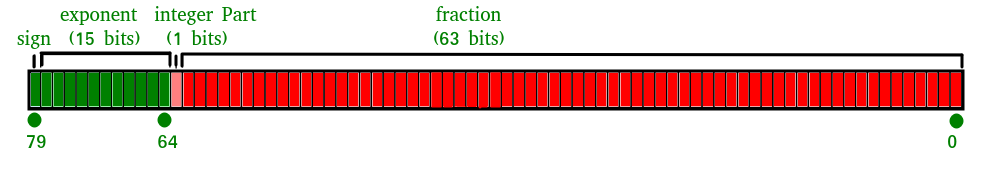# JavaScript Number isSafeInteger() Method

Below is the example of the Number isSafeInteger() Method.

• Example:

 `<``script` `type` `= ``"text/javascript"``>  ` `document.write("Output : " + Number.isSafeInteger(44)); ` ` `

• OUTPUT:
`Output : true`

What is a Safe Integer?
A safe integer is an integer which has the following properties

• A number which can be represented as an IEEE-754 double precision number i.e. all integers from (253 – 1) to -(253 – 1)).

What is an IEEE-754 double-precision number?
Double-precision floating-point format is a computer number format, which occupies 64 bits in computer memory.
It represents a wide range of numeric values by using a floating-point.

The IEEE 754 standard specifies a binary64 as having:

Sign bit: 1 bit
Exponent: 11 bits
Significand precision: 53 bits (52 explicitly stored)isSafeInteger() Method In JavaScript

The isSafeInteger() method in JavaScript is used to check whether a number is a safe integer or not.

Syntax:

`Number.isSafeInteger(Value)`

Parameters Used:
1. Value :It is the number to be check for safeinteger() method.

Return Value:
The toExponential() method in JavaScript returns true if the value is a safe integer Number, else it returns false.

Examples:

```Input : Number.isSafeInteger(23)
Output : true

Input : Number.isSafeInteger(-23)
Output : true

Input : Number.isSafeInteger(0.5)
Output : false

Input : Number.isSafeInteger(0/0)
Output : false

```
1. Passing a positive number as an argument in the isSafeInteger() method.

 `<``script` `type` `= ``"text/javascript"``>  ` `document.write("Output : " + Number.isSafeInteger(23)); ` ` `

OUTPUT:

`Output : true`
2. Passing a negative number as an argument in the isSafeInteger() method.

 `<``script` `type` `= ``"text/javascript"``>  ` `document.write("Output : " + Number.isSafeInteger(-23)); ` ` `

OUTPUT:

`Output : true`
3. Passing a number(with decimals) as an argument in the isSafeInteger() method.

 `<``script` `type` `= ``"text/javascript"``>  ` `document.write("Output : " + Number.isSafeInteger(0.5)); ` ` `

OUTPUT:

`Output : false`
4. Passing an equation( which equates to an infinte value) as an argument in the isSafeInteger() method.

 `<``script` `type` `= ``"text/javascript"``>  ` `document.write("Output : " + Number.isSafeInteger(0 / 0)); ` ` `

OUTPUT:

`Output : false`

Code Explanation:
JavaScript uses double-precision floating-point format numbers as specified in IEEE 754 and can only safely represent numbers between -(253 – 1) and 253 – 1.If the parameter passed lies in this specified range then the number.isSafeInteger() method returns true else false.

Supported Browsers:

• Internet Explorer
• Firefox
• Apple Safari
• OperaMy Personal Notes arrow_drop_upI am a technology enthusiast who has a keen interest in programming I am pursuing Engineering in Computer Science from GEU, Dehradun I like to unwind by watching movies and English sitcomsI have a keen interest in music

If you like GeeksforGeeks and would like to contribute, you can also write an article using contribute.geeksforgeeks.org or mail your article to contribute@geeksforgeeks.org. See your article appearing on the GeeksforGeeks main page and help other Geeks.

Please Improve this article if you find anything incorrect by clicking on the "Improve Article" button below.

Article Tags :

Be the First to upvote.

Please write to us at contribute@geeksforgeeks.org to report any issue with the above content.